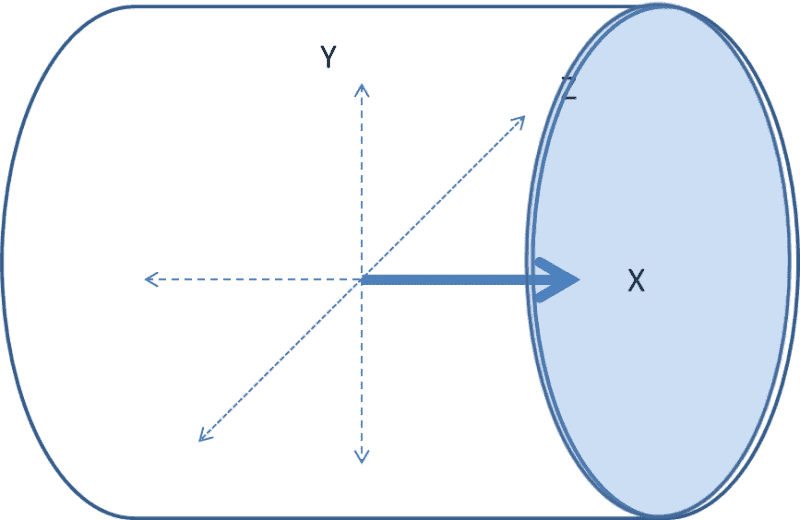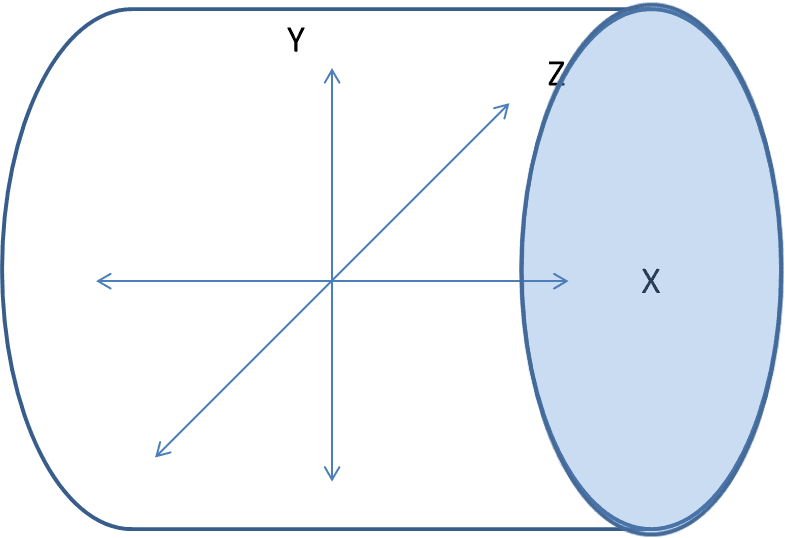# Surge Pressure Stagnation Pressure Static Pressure Bernoulli

I have trouble understanding why Stagnation Pressure equals Total Pressure when we apply Bernoullis theorem to a constriction.
Two different scenarios
Scenario 1.A pipe has pressure $p_1$ , its flow is velocity $v_1$ and a diameter $a_1$ ,it has no restriction along its length.
Total Pressure $Tp_1$ is therefore
$Tp_1 = p_1 + \frac{1}{2}mv^2$

Scenario 2. A pipe pressure $p_1$, initial velocity of $v_1$ ,initially has a diameter $10a_1$, it is then restricted to the same diameter $a_1$ as in scenario 1 . According to Bernoullis its velocity thru this restriction increases to $10v_1$, and its pressure drops to $p_2 = Tp - \frac{1}{2}m.10v^2$
Total Pressure $Tp_1$ is therefore,

Before constriction
$Tp_1 = p_1 + \frac{1}{2}m.v^2$

within constriction
$Tp_1 = p_2 + \frac{1}{2}m.10v^2$
$p_2 = Tp_1 - \frac{1}{2}m.10v^2$

In both examples Total Pressure $Tp_1$ or Stagnation Pressure are equivalent .

I agree that, over time, the pressure will normalise to the same value but how can this be correct instantaneously ? Wouldn't scenario 2 exert an initially higher stagnation pressure (Than in Scenario 1 and Total pressure) against the obstruction and take fractionally longer to normalise ?

Reasoning:

Energy Mass have been conserved by transferring some scalar random particle velocity (hydrostatic pressure) to vector kinetic energy (Dynamic Pressure)

In the second scenario the kinetic energy of the first particles initially hitting the obstruction is 10x times greater due to their velocity in one degree of freedom along the streamline, whereas the hydrostatic pressure reduction must be shared amongst all degrees of freedom at this point and thus wouldn't fully compensate

I liken it to Surge Pressure/Water Hammer in a tube but the explanations I have seen explain this by way of the mass/energy of all of the particles 'piling up' behind the obstruction explaining the shockwave but I am thinking the increase in velocity will also contribute a initial pressure spike above static pressure .

Thanks for the post! This is an automated courtesy bump. Sorry you aren't generating responses at the moment. Do you have any further information, come to any new conclusions or is it possible to reword the post?

Bernoulli eqs. apply to ideal fluids in stationary flow. When you suddently change velocity/impulse/pressure at one place, disturbances propagate at speed of sound in fluid and other equations must be applied.

I have trouble understanding why Stagnation Pressure equals Total Pressure

In this case they are the same simply because they both have the same definition. I believe they are only different if you also include the effect of gravitational head.

The stagnation pressure is a property of the fluid at a given point and is defined as the static pressure that would be obtained if the fluid were isentropically brought to rest and all of the kinetic energy were converted to potential energy as static pressure. And total pressure is simply the sum of static pressure and dynamic pressure (in other words it is the total energy). So the two must be equivalent. Honestly I am a little confused by your question.

You should not use 1/2 * m * V^2 as m is used to refer to mass and dynamic pressure is 1/2 * density * V^2.

Bernoulli eqs. apply to ideal fluids in stationary flow. When you suddently change velocity/impulse/pressure at one place, disturbances propagate at speed of sound in fluid and other equations must be applied.

Yes this is what I am interested in. Can you please expand on what these other equations are or direct me to something that does ?

Honestly I am a little confused by your question.

An attempt at rephrasing the question.

I know this "disturbance" propogates at the speed of sound but at very small time scales - what is the mechanism that transfers this directional kinetic energy (dynamic pressure) into random kinetic energy (Static pressure.)

I would expect the obstruction placed ahead of the flow to initially experience a force over its area many times greater than the eventual static pressure (Force/Area) because this higher kinetic energy/velocity along one streamline /degree of freedom must be spread randomly over all degrees of freedom in the fluid and the velocity averaged to abide by Pascals Law. Am I correct ?

You should not use 1/2 * m * V^2 as m is used to refer to mass and dynamic pressure is 1/2 * density * V^2.

I understood that these are interchangeable? I use mass as I am trying to understand this concept from Newton's Laws of motion not conservation laws.

Ugh the Navier Stokes I was hoping to avoid this as my Calc is deficient. If I could borrow a little from it and present in a graphical format to obtain a more qualitative understanding rather than a proof ? To restate -

• I understand that I can take any fluid volume along a streamline and the energy contained will be constant
• I understand from Bernoullis that these can change energy between Dynamic Pressure Static Pressure and PE
• For a unchanging height datum this reduces to Dynamic Pressure + Static pressure = Constant (I take this to mean that the Sum of all molecular velocities is Constant )

Question
How can the force applied in the X direction against the blue plate surface be equal between these scenarios representing Stagnation Pressure and Static Pressure?

1. A flow (due to a constriction) has converted some energy from Static pressure (diminishment represented by Y,Z,-X,-Y,-Z dotted lines) to Dynamic Pressure ( increase represented by thickened solid X line ).This flow is then instaneously halted by the blue plate surface. (Stagnation Pressure)2. Momentarily later, as the flow is stopped, that same energy constant is applied (according to Pascals) equally in all degrees of freedom within that volume ( represented by equal X,Y,Z,-X,-Y,-Z lines) (Static Pressure)I cannot see how these equal ?

You don't need full form Navier-Stokes for such problems at all. Looks like you didn't read the pdf I provided... Particularly chapters 1.7. and 1.9.
And concerning subclasses of Euler equations in fluid dynamics you can also read about on the wiki page.